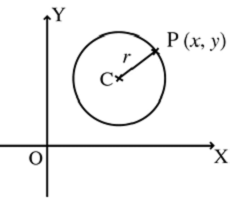# Equation of a Circle

A circle is the locus of a point which moves in such a way that its distance from a fixed point is always constant. The fixed point is called the centre of the circle and the constant distance is called the radius of the circle.

Let C(h, k) be the centre and r be the radius of the circle. Let P(x, y) be any point on the circle.CP = r

CP2 = r2

(x − h)2 + (y−k)2 = r2

If the centre of the circle is at the origin, (h, k) = (0, 0) then the equation of the circle is

x2 + y2 = r2

The equation of a circle if the end points of a diameter are given

Let A(x1, y1) and B(x2, y2) be the end points of a diameter. Then, the equation of the circle is

(x − x1)(x − x2) + (y − y1)(y − y2) = 0